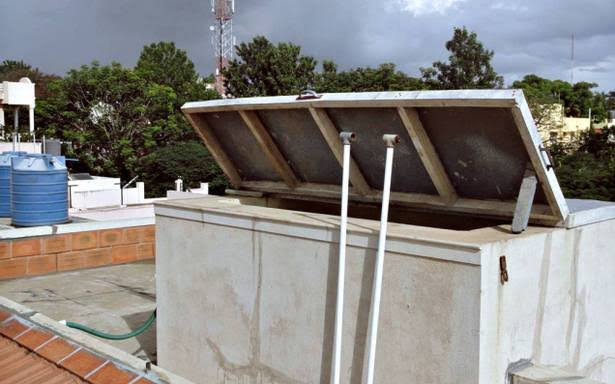# Water-Sump/Tank CalculatorIS 3370-1

##Water-Sump/Tank Calculation

meter
cm
meter
cm
meter
cm

#### Volume = 1620.00 m3 | 57209.81 ft3

Water-sump/Tank Calculation

Total Volume = ${\mathrm{Length}}_{}×{\mathrm{Width}}_{}×{\mathrm{Depth}}_{}$

Total Volume = ${\mathrm{12}}_{}×{9}_{}×{\mathrm{15}}_{}$

Total Volume = 1620.00 m3

Total Quantity = ${\mathrm{Total Volume}}_{}×{\mathrm{1000}}_{}$

Total Quantity = ${\mathrm{1620.0000}}_{}×{\mathrm{1000}}_{}$

Total Quantity = 1620000.00 lt

## What is water-sump/tank calculation?

A water tank is a container for storing water.

Water tanks are used to provide storage of water for use in many applications, drinking water, irrigation agriculture, fire suppression, agricultural farming, both for plants and livestock, chemical manufacturing, food preparation as well as many other uses.

A lot of variety exists in water tank and there are different types of tank:

• Chemical contact tank
• Ground water tank
• Elevated water tank##### Water-Sump/Tank calculation
${\mathrm{Volume of Water-Sump/Tank =}}_{}{\mathrm{Length}}_{}×{\mathrm{Width}}_{}×{\mathrm{Depth}}_{}$

${\mathrm{Total Quantity in Liter\left(lt.\right) =}}_{}{\mathrm{Volume of Water-Sump/Tank}}_{}×{\mathrm{1000}}_{}$
Where,
• m3 (Cubic meter) and ft3 (Cubic feet) is a total volume and Lt (liter) is a total volume in liter.
• Length, breadth, and depth in meter/cm and total volume in Lt (liter).

Note:
1 m3 = 35.3147 ft3## Importance of water-sump/tank

CA water tank or container should do no harm to the water. Water is susceptible to a number of ambient negative influences, including bacteria, viruses, algae, changes in pH, and accumulation of minerals, accumulated gas. The contamination can come from a variety of origins including piping, tank construction materials, animal and bird feces, mineral and gas intrusion. A correctly designed water tank works to address and mitigate these negative effects.It is imperative that water tanks be cleaned annually to preclude delivery of algae, bacteria and viruses to people or animals.